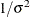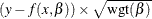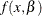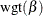### Special Variables

Several special variables are created automatically and can be used in PROC NLIN programming statements.

#### Special Variables with Values That Are Set by PROC NLIN

The values of the following six special variables are set by PROC NLIN and should not be reset to a different value by programming statements:

_ERROR_

is set to 1 if a numerical error or invalid argument to a function occurs during the current execution of the program. It is reset to 0 before each new execution.

_ITER_

represents the current iteration number. The variable _ITER_ is set to –1 during the grid search phase.

_MODEL_

is set to 1 for passes through the data when only the predicted values are needed, and not the derivatives. It is 0 when both predicted values and derivatives are needed. If your derivative calculations consume a lot of time, you can save resources by using the following statement after your MODEL statement but before your derivative calculations:

if _model_ then return;


The derivatives generated by PROC NLIN do this automatically.

_N_

indicates the number of times the PROC NLIN step has been executed. It is never reset for successive passes through the data set.

_OBS_

indicates the observation number in the data set for the current program execution. It is reset to 1 to start each pass through the data set (unlike the _N_ variable).

_SSE_

contains the error sum of squares of the last iteration. During the grid search phase, the _SSE_ variable is set to 0. For iteration 0, the _SSE_ variable is set to the SSE associated with the point chosen from the grid search.

_P_WEIGHTED_SSE_

contains the error sum of squares for the optimization tasks that are required for profiling parameters and assessing the influence of each observation on the estimate of each profiled parameter.

#### Special Variable Used to Determine Convergence Criteria

The special variable _LOSS_ can be used to determine the criterion function for convergence and step shortening. PROC NLIN looks for the variable _LOSS_ in the programming statements and, if it is defined, uses the (weighted) sum of this value instead of the residual sum of squares to determine the criterion function for convergence and step shortening. This feature is useful in certain types of maximum-likelihood estimation.

Note: Even if you specify the _LOSS_ variable in your programming statements, the NLIN procedure continues to solve a least squares problem. The specified _LOSS_ variable does not define or alter the objective function for parameter estimation.

#### Weighted Regression with the Special Variable _WEIGHT_

To obtain weighted nonlinear least squares estimates of parameters, make an assignment to the _WEIGHT_ variable as in the following statement:

_weight_ = expression;


When this statement is included, the expression on the right side of the assignment statement is evaluated for each observation in the data set. The values multiplied byare then taken as inverse elements of the diagonal variance-covariance matrix of the dependent variable.

When a variable name is given after the equal sign, the values of the variable are taken as the inverse elements of the variance-covariance matrix. The larger the _WEIGHT_ value, the more importance the observation is given.

The _WEIGHT_ variable can be a function of the estimated parameters. For estimation purposes, the derivative of the _WEIGHT_ variable with respect to the parameters is not included in the gradient and the Hessian of the loss function. This is normally the desired approach for iteratively reweighted least squares estimation. When the _WEIGHT_ variable is a function of the parameters, the gradient and the Hessian used can lead to poor convergence or nonconvergence of the requested estimation. To have the derivative of the _WEIGHT_ variable with respect to the parameters included in the gradient and the Hessian of the loss function, do not use the _WEIGHT_ variable. Instead, redefine the model aswhere y is the original dependent variable,is the nonlinear model, andis the weight that is a function of the parameters.

If the _WEIGHT_= statement is not used, the default value of 1 is used, and regular least squares estimates are obtained.